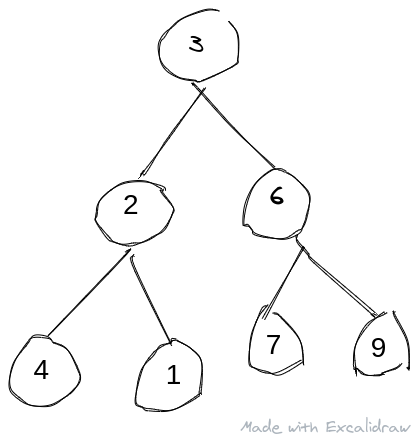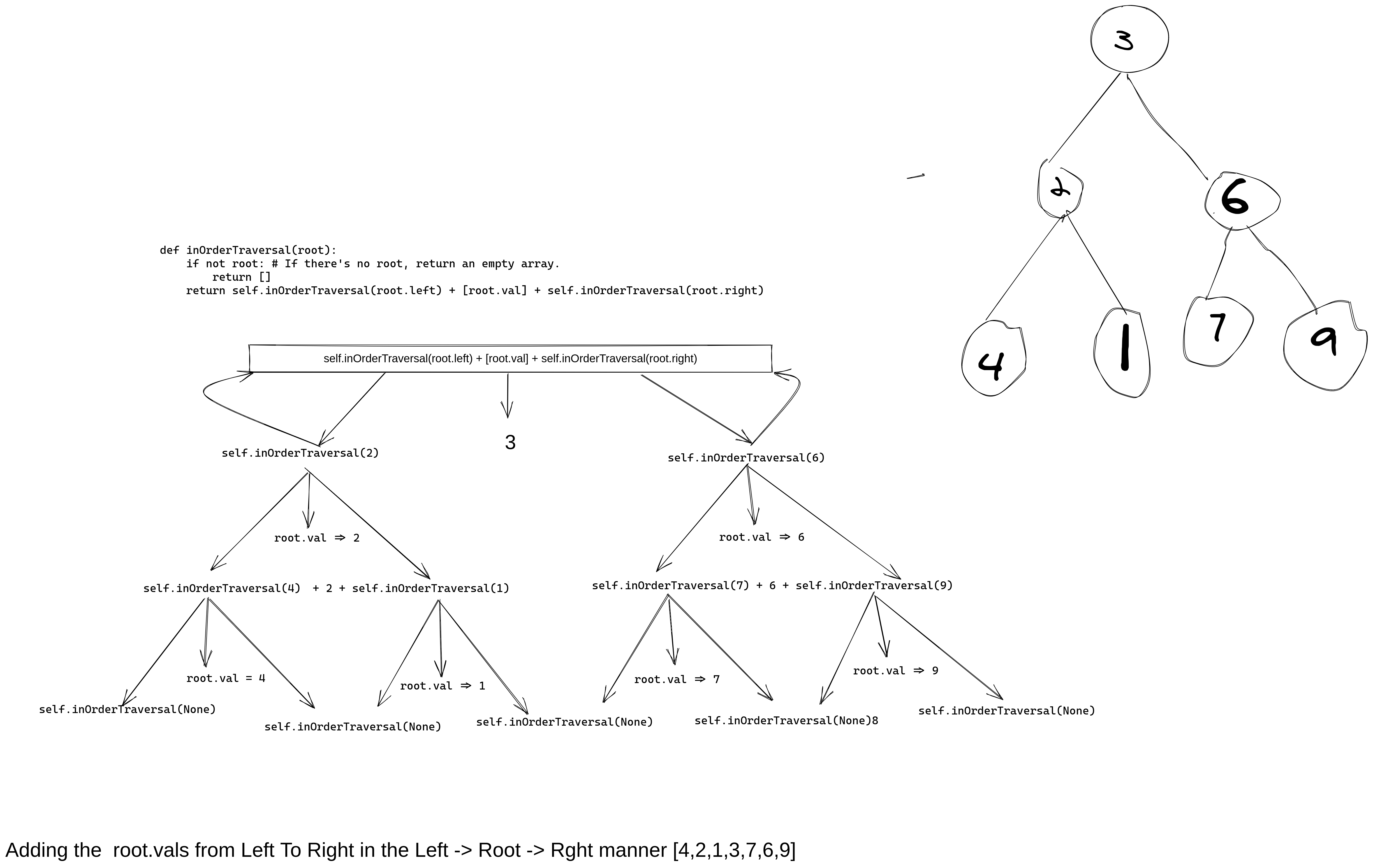I don't know it all, I might be wrong, If I'm wrong, please correct me.

## Exploring Recursion

A simple binary tree displayed below, and I'll be trying to explain recursion with it.

Say the code for our tree node is:

TreeNode.py
``````class TreeNode:
def __init__(self, val = 0, left = None, right = None):
self.val = val
self.left = left
self.right = right``````

So for the awkwardly drawn tree ( I no sabi draw like that ), we have 7 nodes in total: A root node, 3, child, 2 and 6, and their offspring, 4, 1, 7, 9 ( You sha get?).An inorder traversal is carried out easily using recursion...

The code for that is:

InorderTraversal.py
``````def inOrderTraversal(root):
if not root: # If there's no root, return an empty array.
return []
return self.inOrderTraversal(root.left) + [root.val] + self.inOrderTraversal(root.right)``````

Wait, what? Just how many lines of code? That's recursion for you..

However, you can also traverse this tree iteratively. But, who likes stress? Not me sha.

So what this code does is it traverse every parent node from left to root to right i.e, our result is => `[4,2,1,3,7,6,9]`

Think of it like this because to be honest, I think this is how it works:

``````- We have a stack, [],then we append only the value of root node passed into it.
That's all.``````

## What?

Yes, the recursive call splits the tree into left and right. Remember that every object of the tree is a node, and a node has left and right nodes as its leaves. So, when the recursive call kicks in on a node, it adds that nodes' value to the stack...

A nodes' left value is prepended to the value of the node, and the right appended to it. This continues till there are no nodes left, only then, will the stack from the left be prepended to the ancestor node value, and the stack from the right appended to the ancestor node.

### But, we should have [[], []]

Haha, nope. The values are added to the main stack as they leave the recursion stack frame in the LIFO manner.

Here's a diagrammatic representation which is also my first time using Excalidraw..## Teinz

I'd like to get feedback and correction on this. Tbh, explaining this in words is hard I won't lie, in person is the best where I can put gra gra lmao...

Wait, I'm talking to my sef. Haha!